# NCERT Solution (Ex 1.2) - Chapter 1: Rational Number , Maths, Class 8 Notes - Class 8

## Class 8: NCERT Solution (Ex 1.2) - Chapter 1: Rational Number , Maths, Class 8 Notes - Class 8

The document NCERT Solution (Ex 1.2) - Chapter 1: Rational Number , Maths, Class 8 Notes - Class 8 is a part of Class 8 category.
All you need of Class 8 at this link: Class 8

Exercise 1.2

Question 1:
Represent these numbers on the number line:
(i)  7/4
(ii) -5/6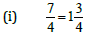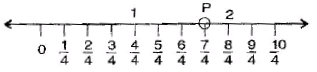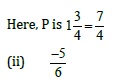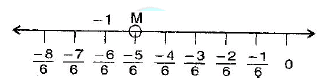Here, M is - 5/6

Question 2:
Represent
-2/11, -5/11. -9/11 on the number line.

Here B=  -2/11, C=-5/11 and D= -9/11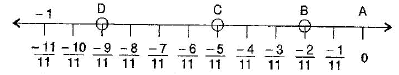Question 3:
Write five rational numbers which are smaller than 2.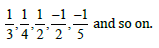Question 4:
Find ten rational numbers between -2/5 and 1/2

Given rational numbers -2/5 and  1/2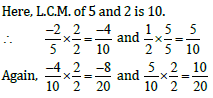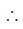Ten rational number between -2/5 and  1/2 are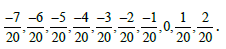Question 5:
Find five rational numbers between:

(i)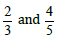(ii)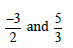(iii)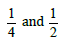(i)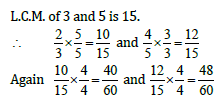Five rational numbers between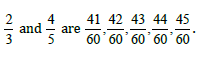(ii)L.C.M. of 2 and 3 is 6.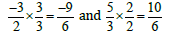Five rational numbers between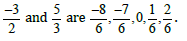(iii)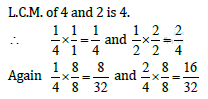Five rational numbers between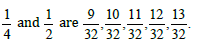Question 6:
Write 5 rational numbers greater than -2.

Five rational numbers greater than -2 are: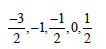[Other rational numbers may also be possible]

Question 7:
Find ten rational numbers between  3/5 and 3/4

The given rational numbers  3/5 and 3/4

L.C.M. of 5 and 4 is 20.Five rational numbers betweenc 3/5 and 3/4 are: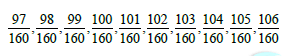The document NCERT Solution (Ex 1.2) - Chapter 1: Rational Number , Maths, Class 8 Notes - Class 8 is a part of Class 8 category.
All you need of Class 8 at this link: Class 8Use Code STAYHOME200 and get INR 200 additional OFF

Track your progress, build streaks, highlight & save important lessons and more!

,

,

,

,

,

,

,

,

,

,

,

,

,

,

,

,

,

,

,

,

,

,

,

,

,

,

,

;